# 分形曼德布罗特集## 朱莉娅集

xn=xn12+\${complex(c)}
x0=\${complex(x0)}
x1=\${complex(x1)}
x2=\${complex(x2)}
x3=\${complex(x3)}

c=0.701760.3842i

c=0.4+0.6i

c=0.285+0.01i

## 曼德布罗特集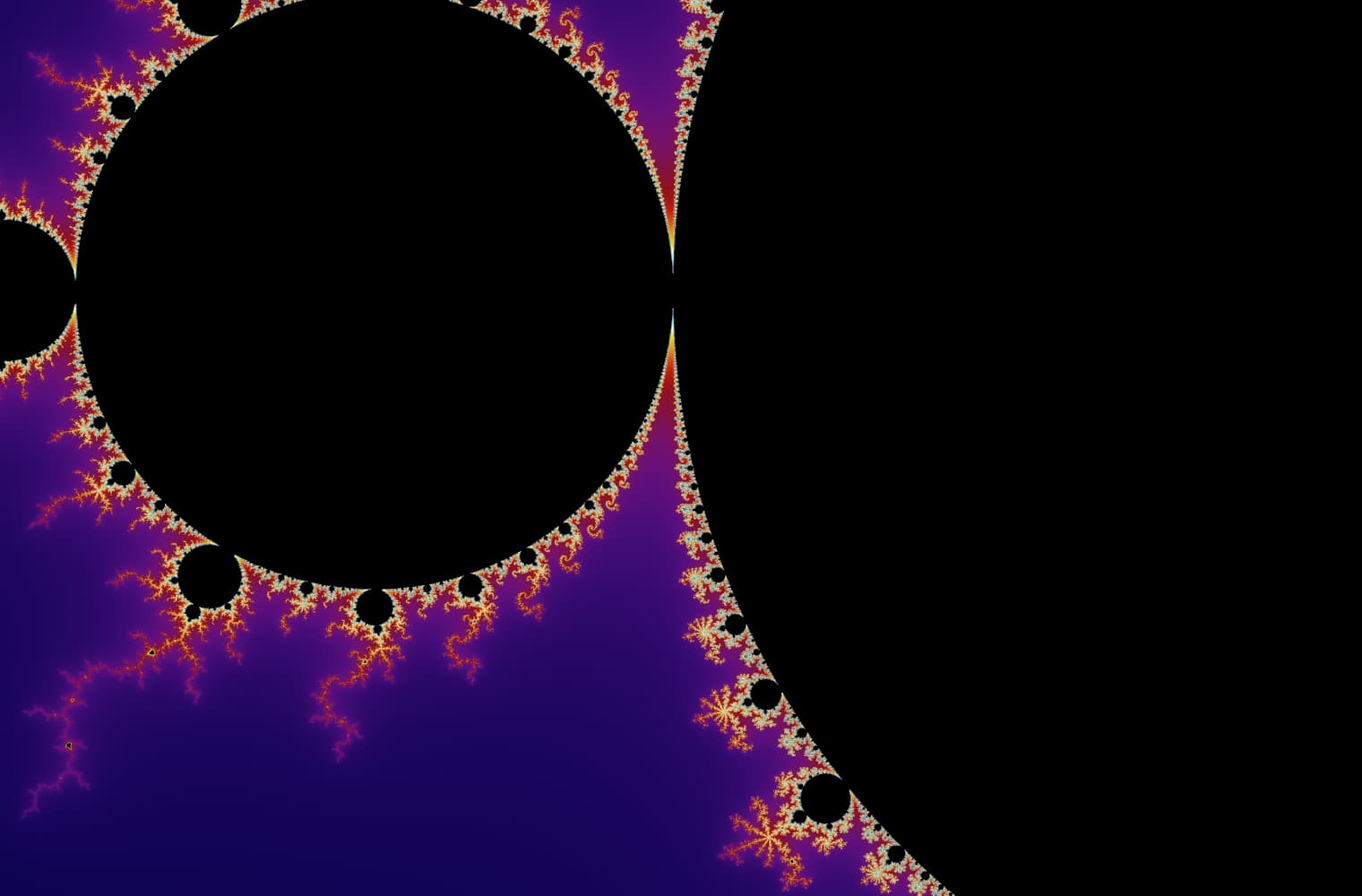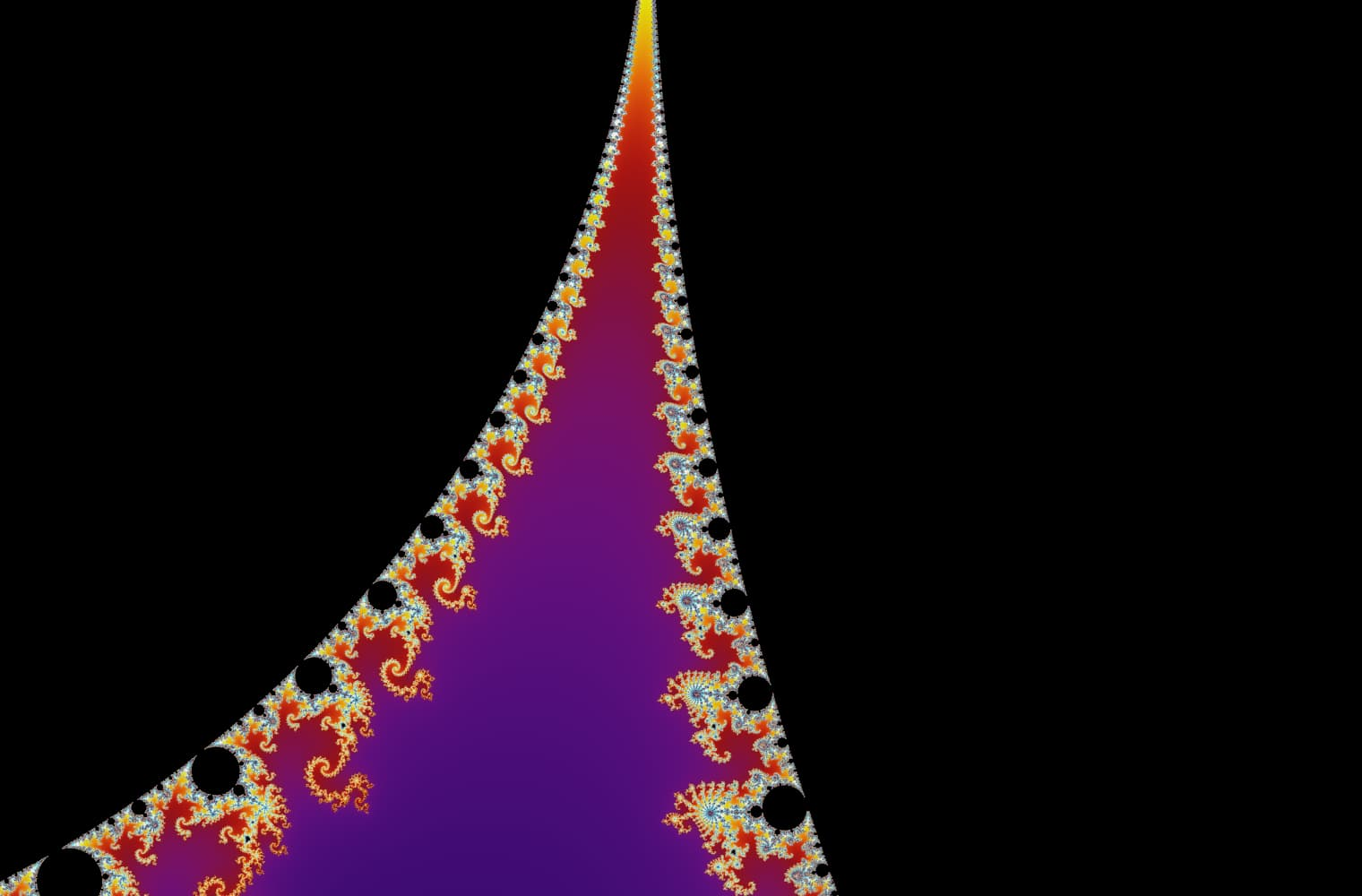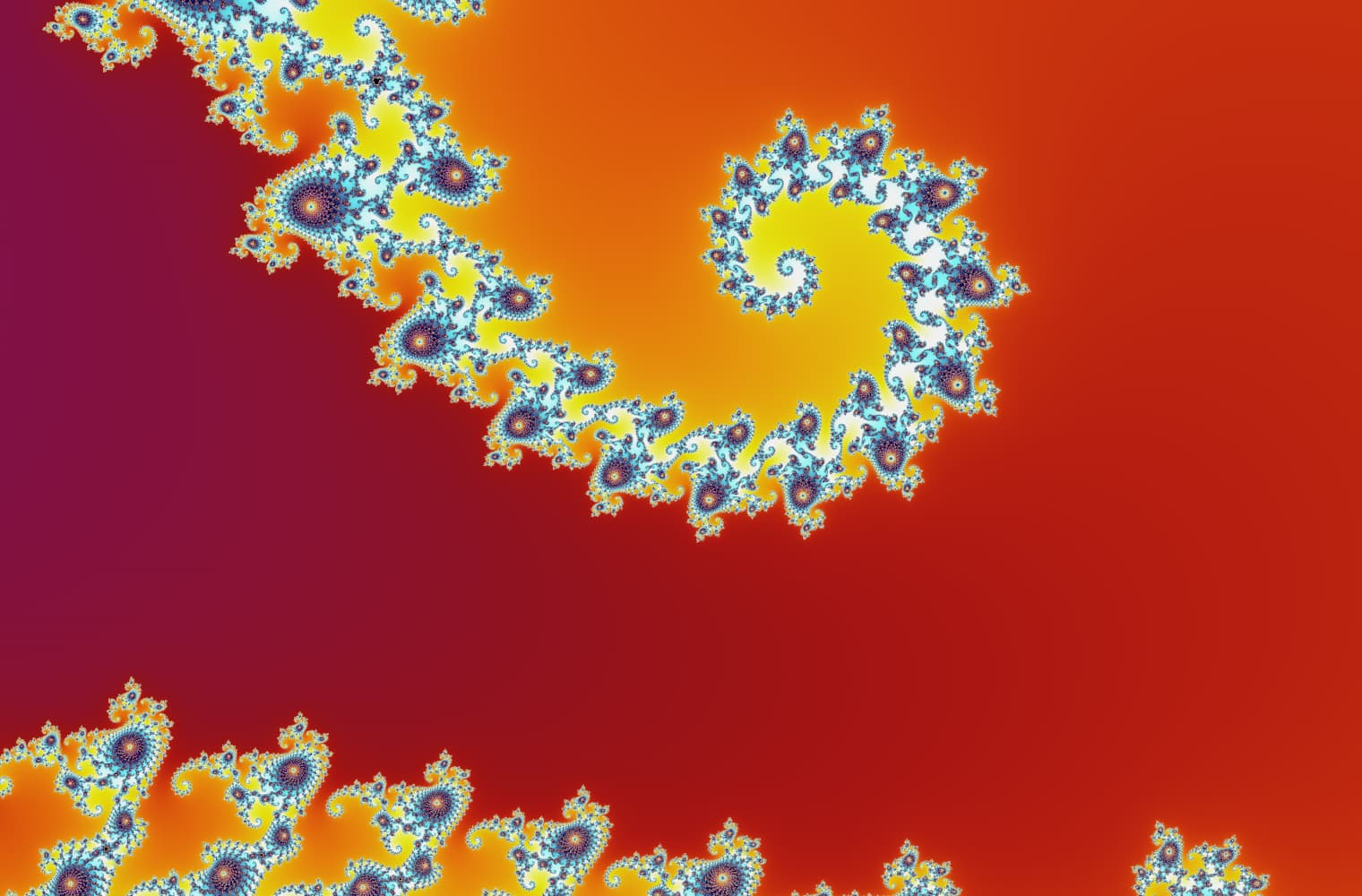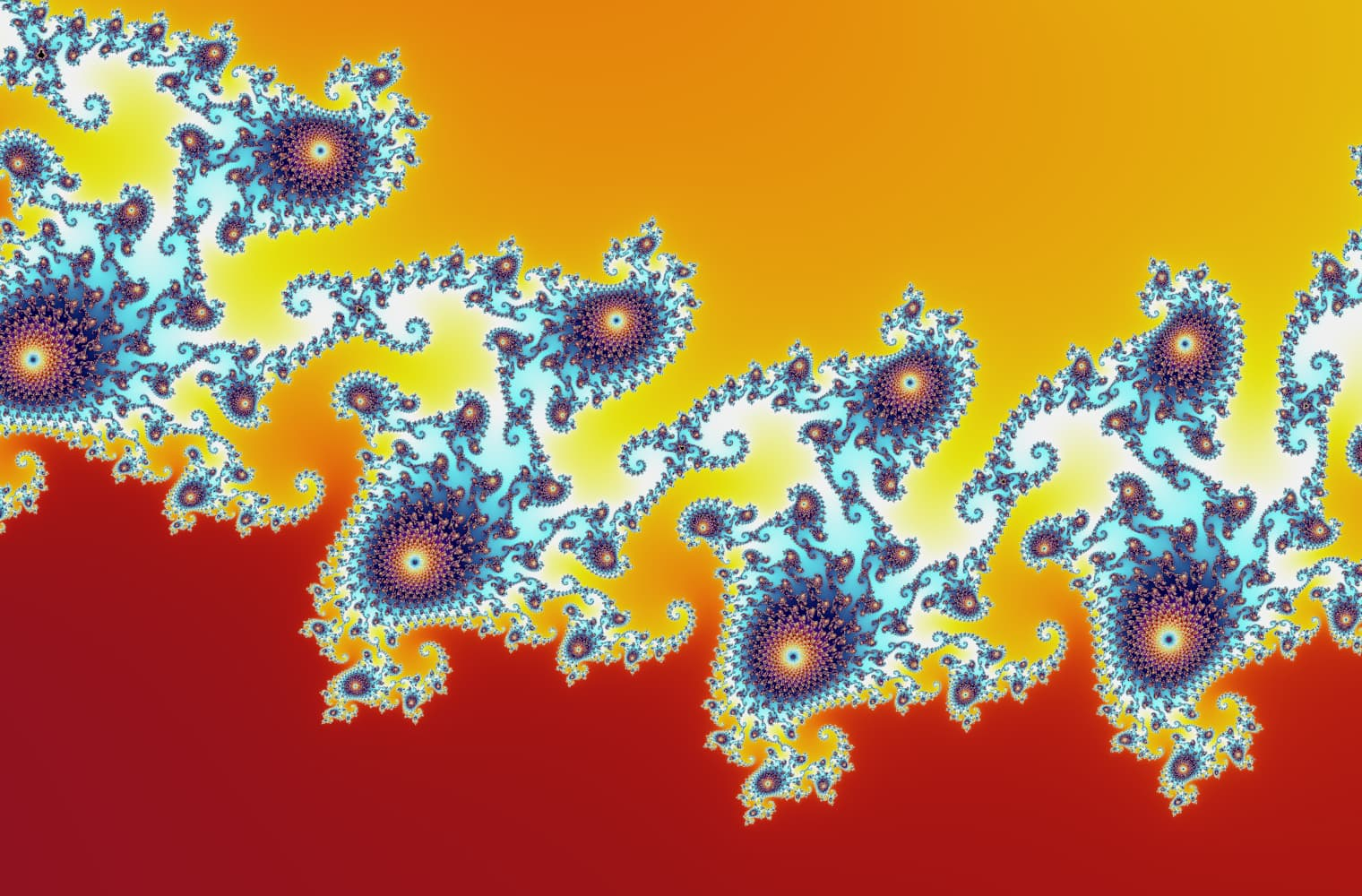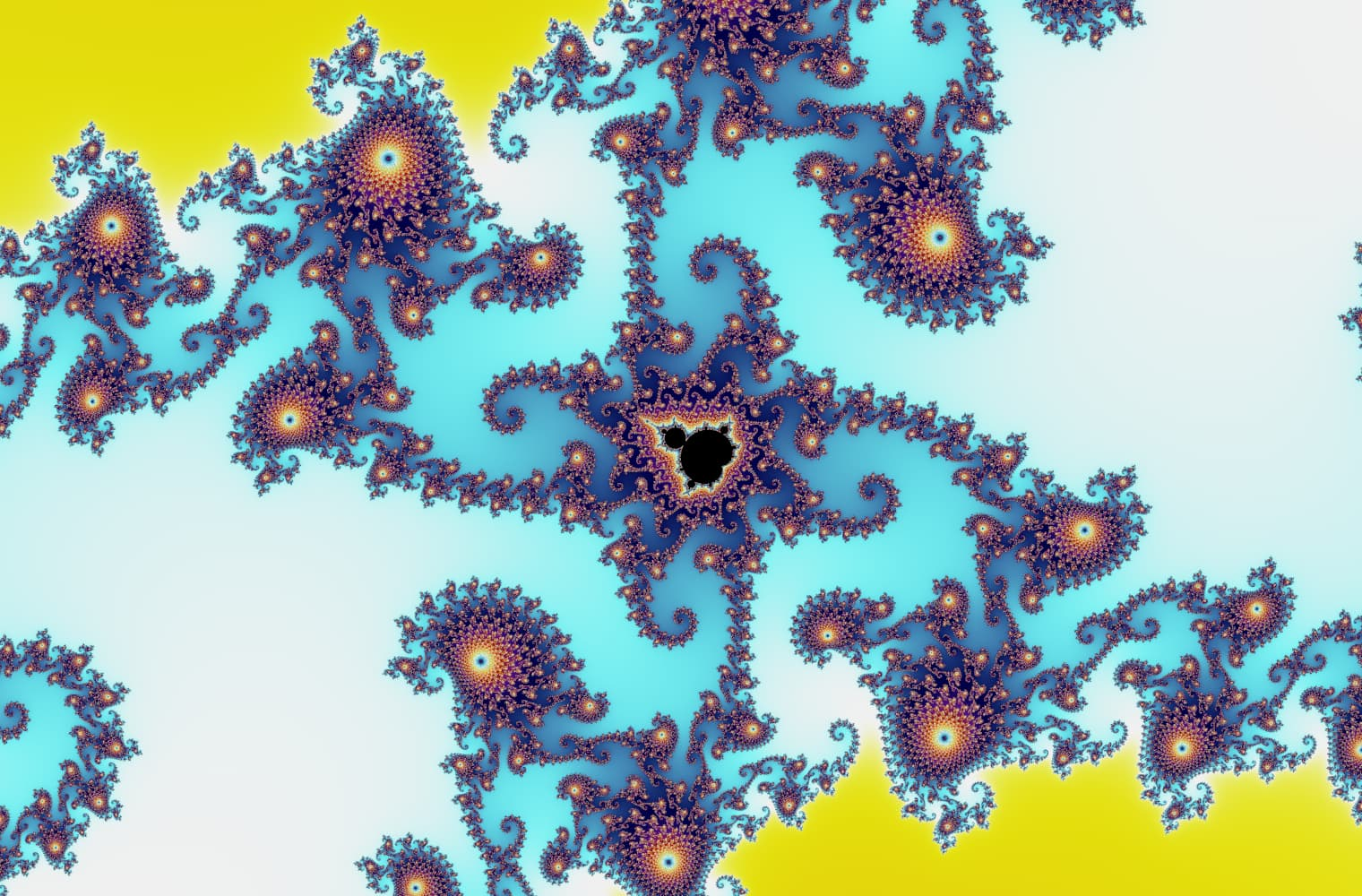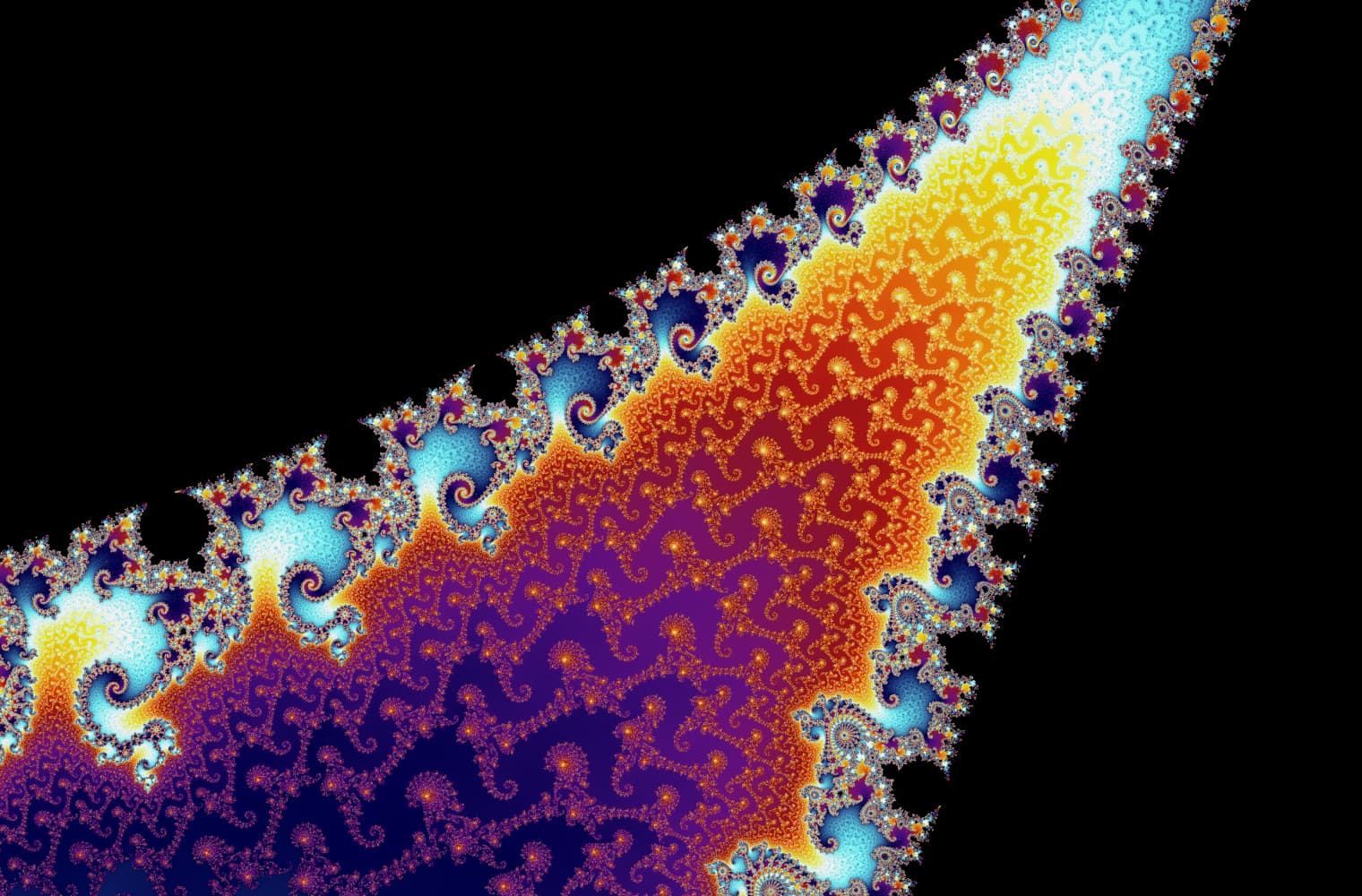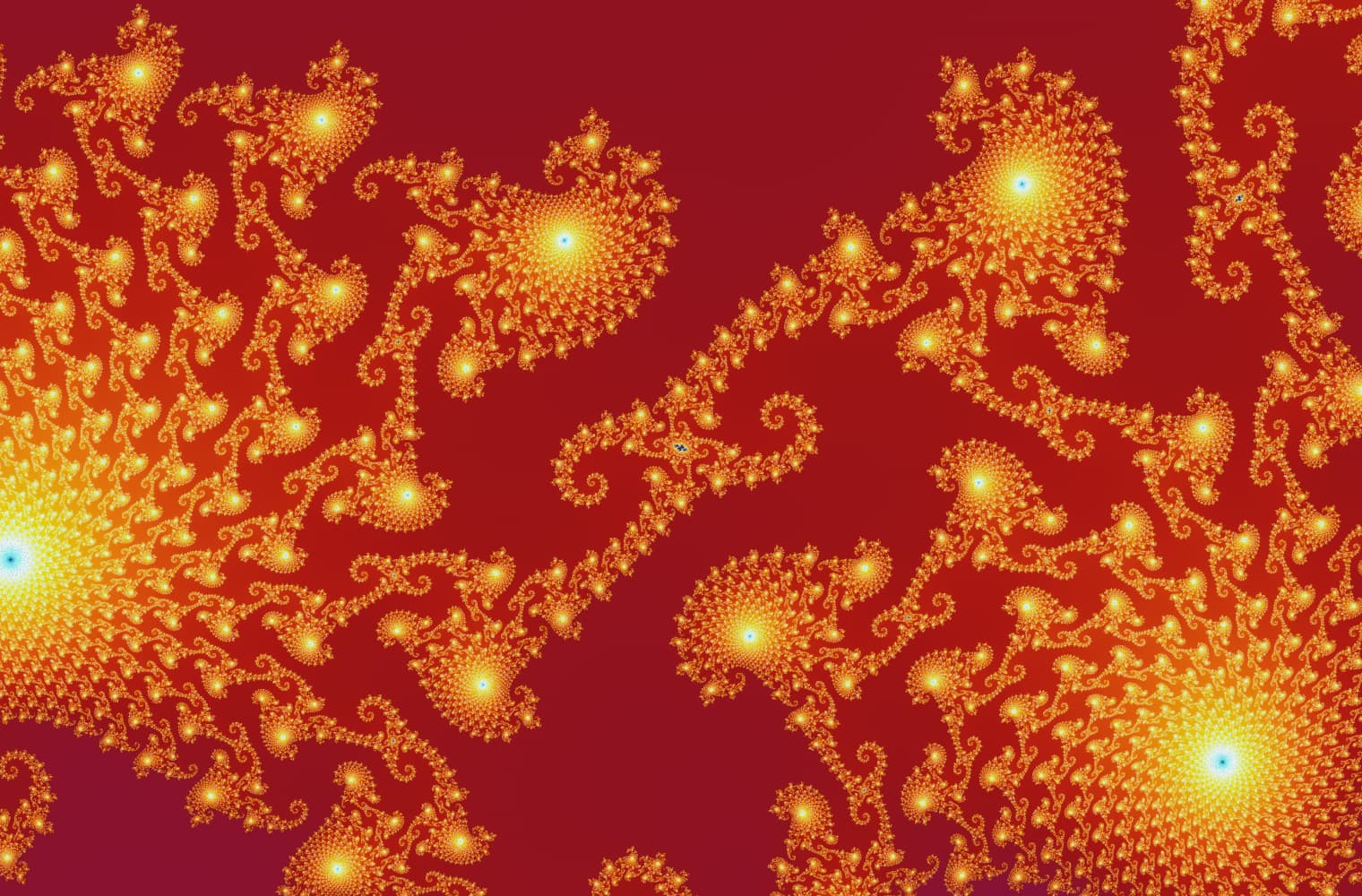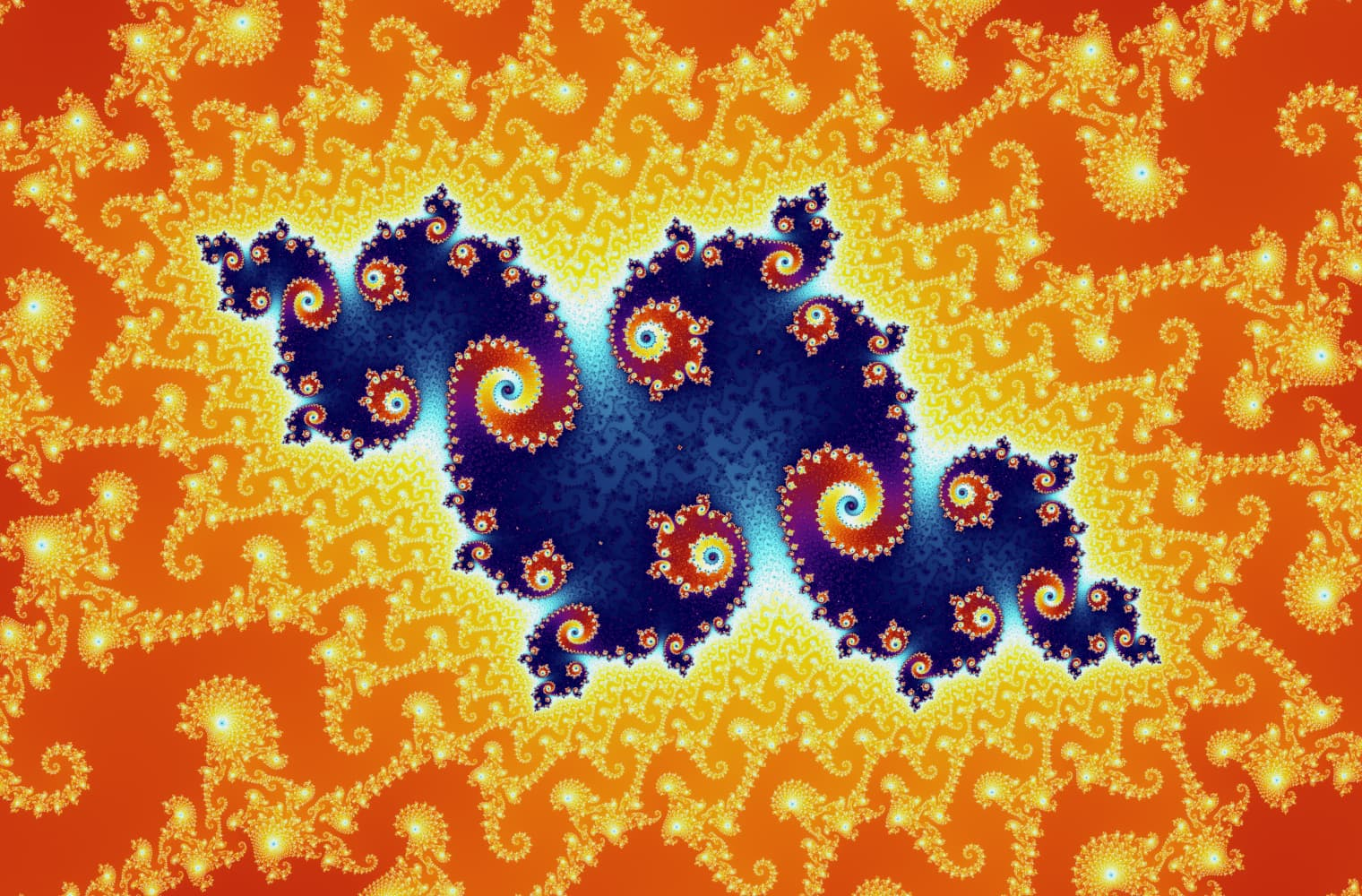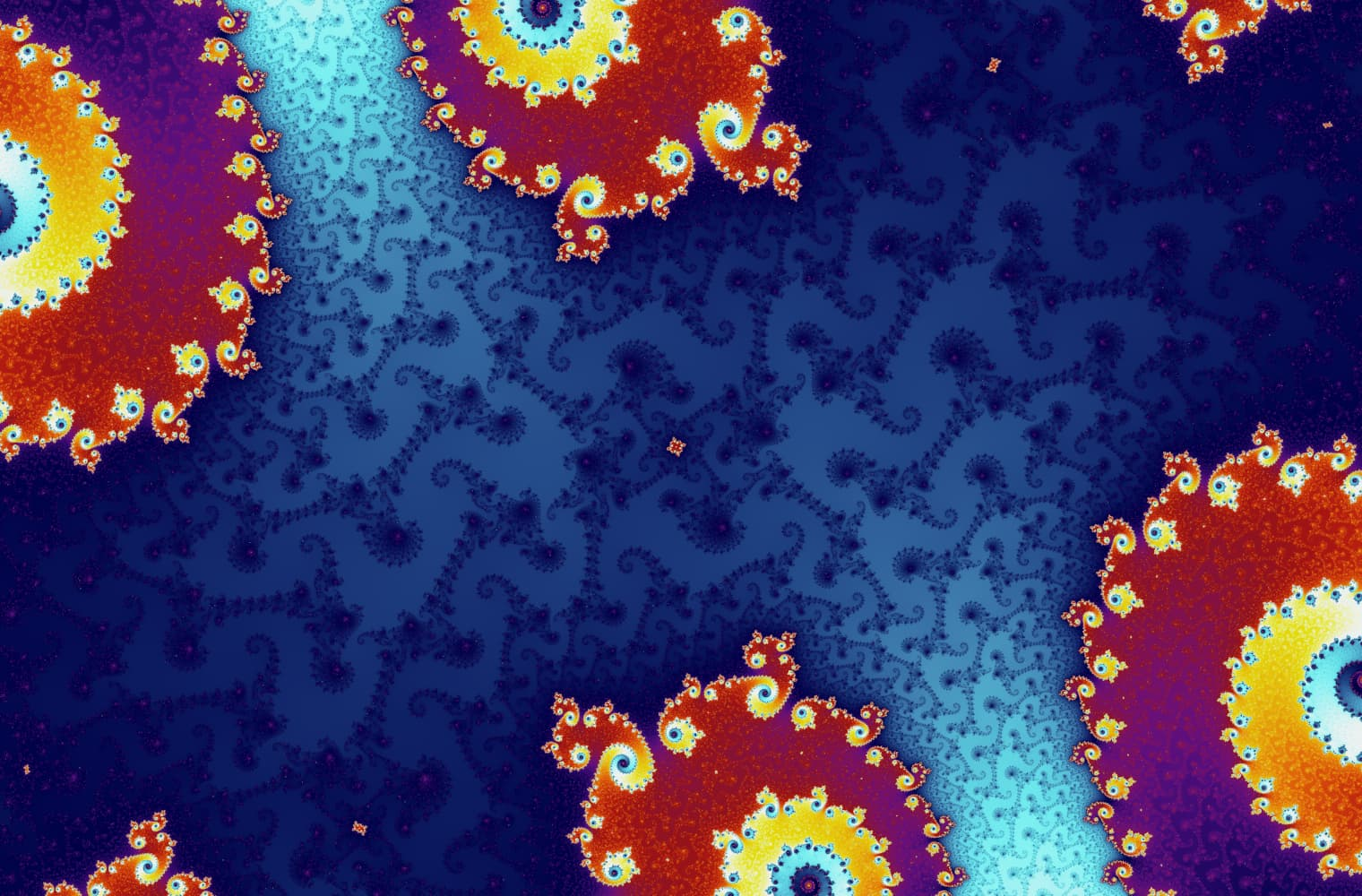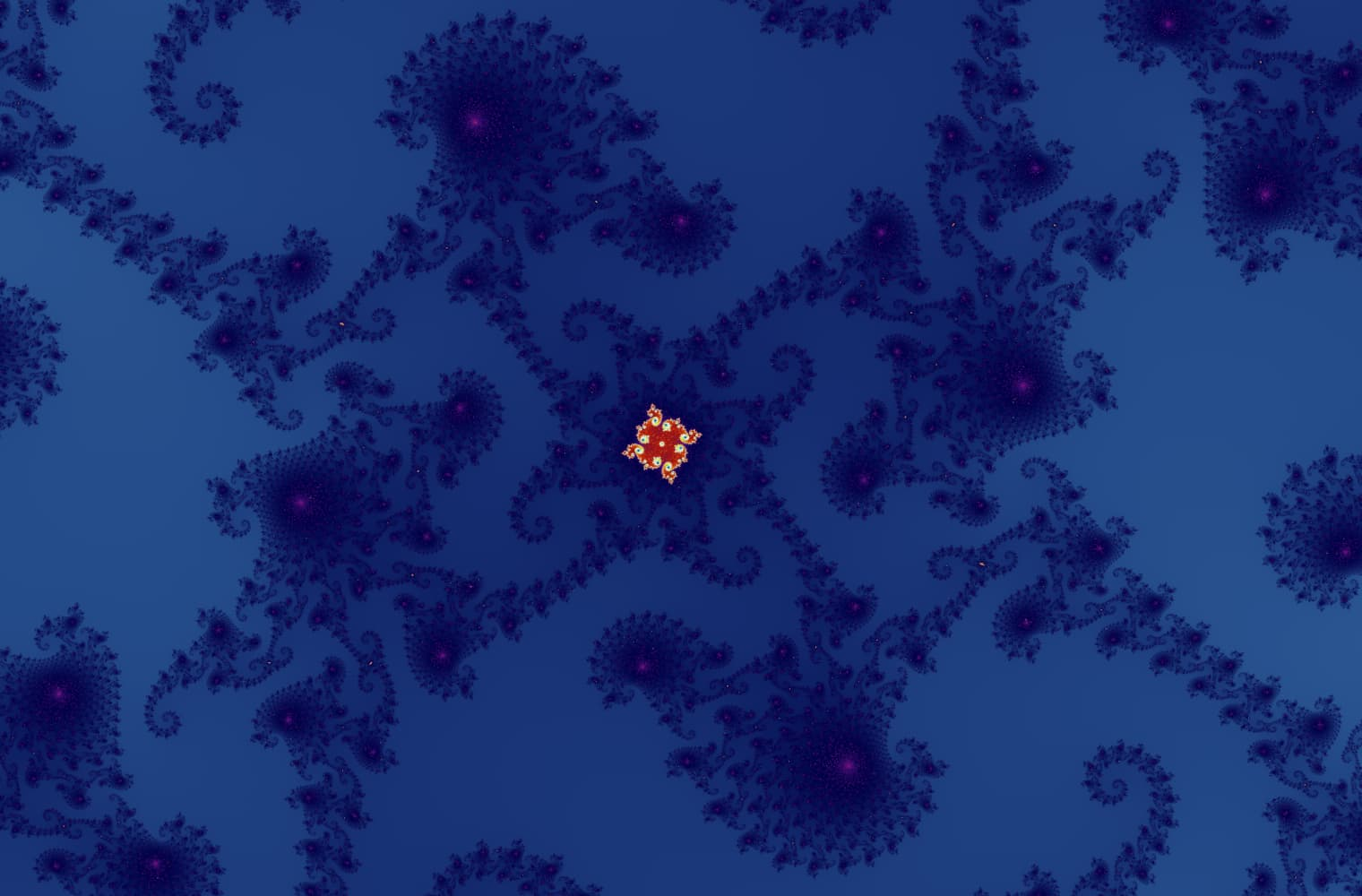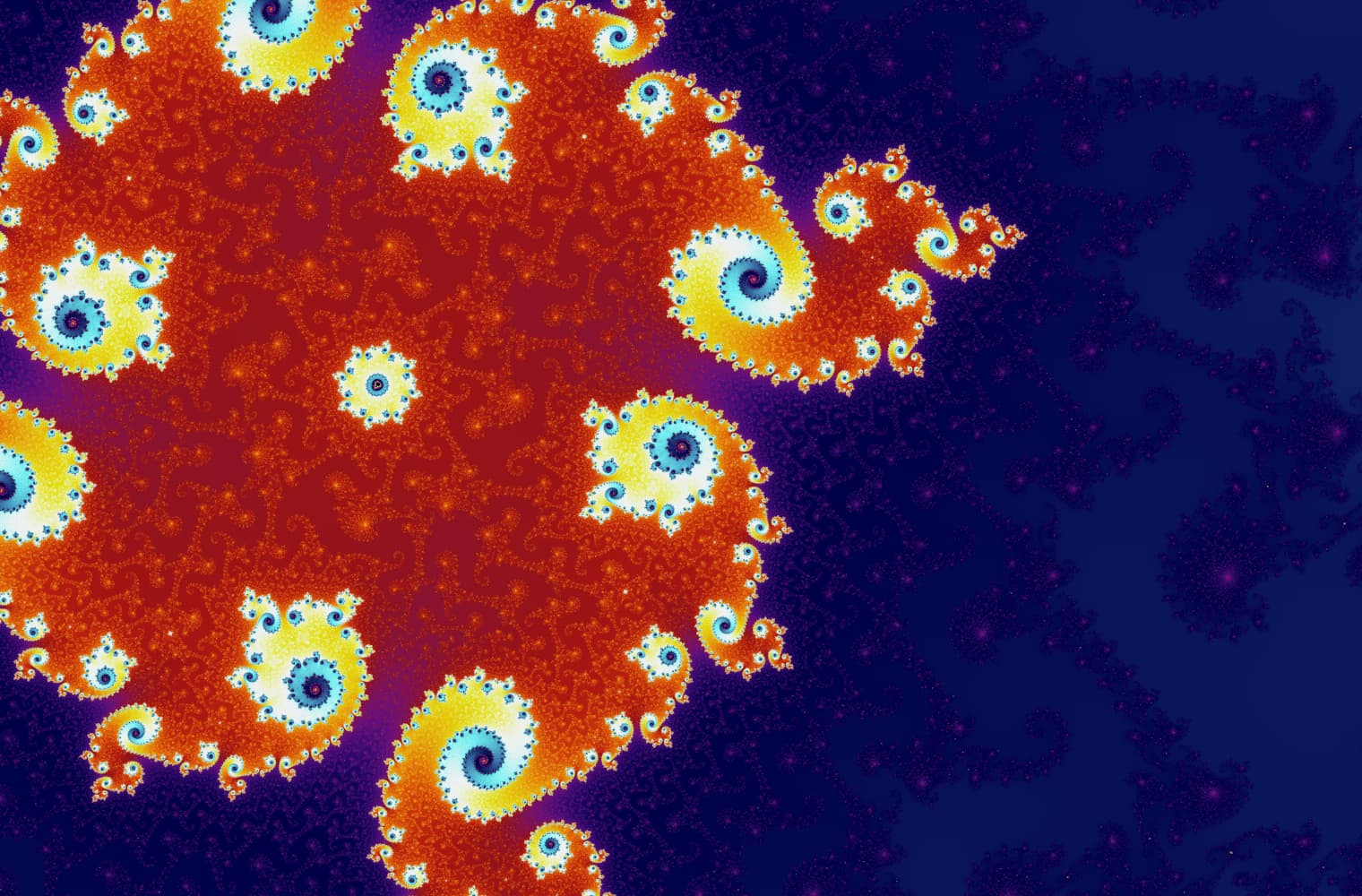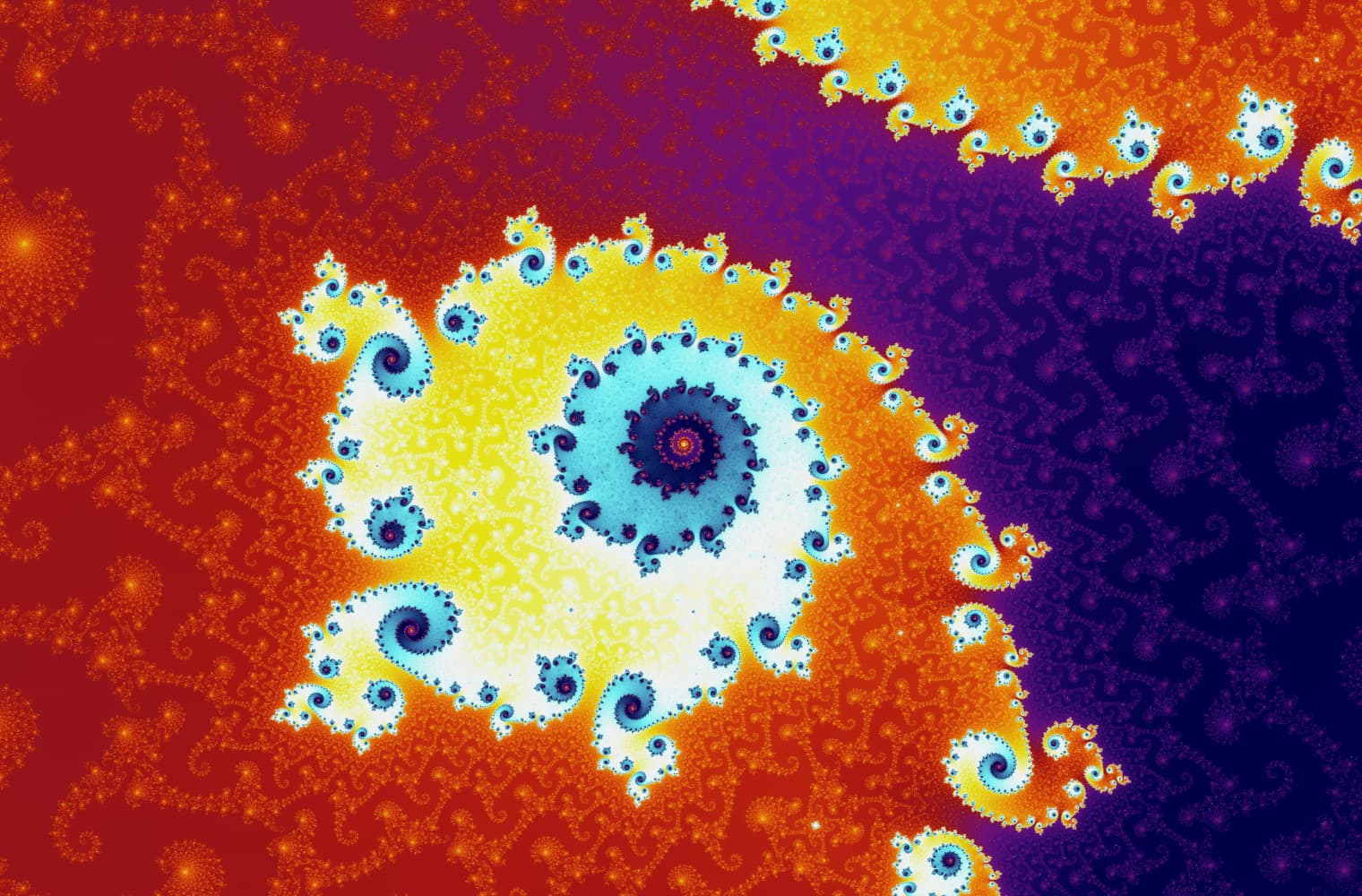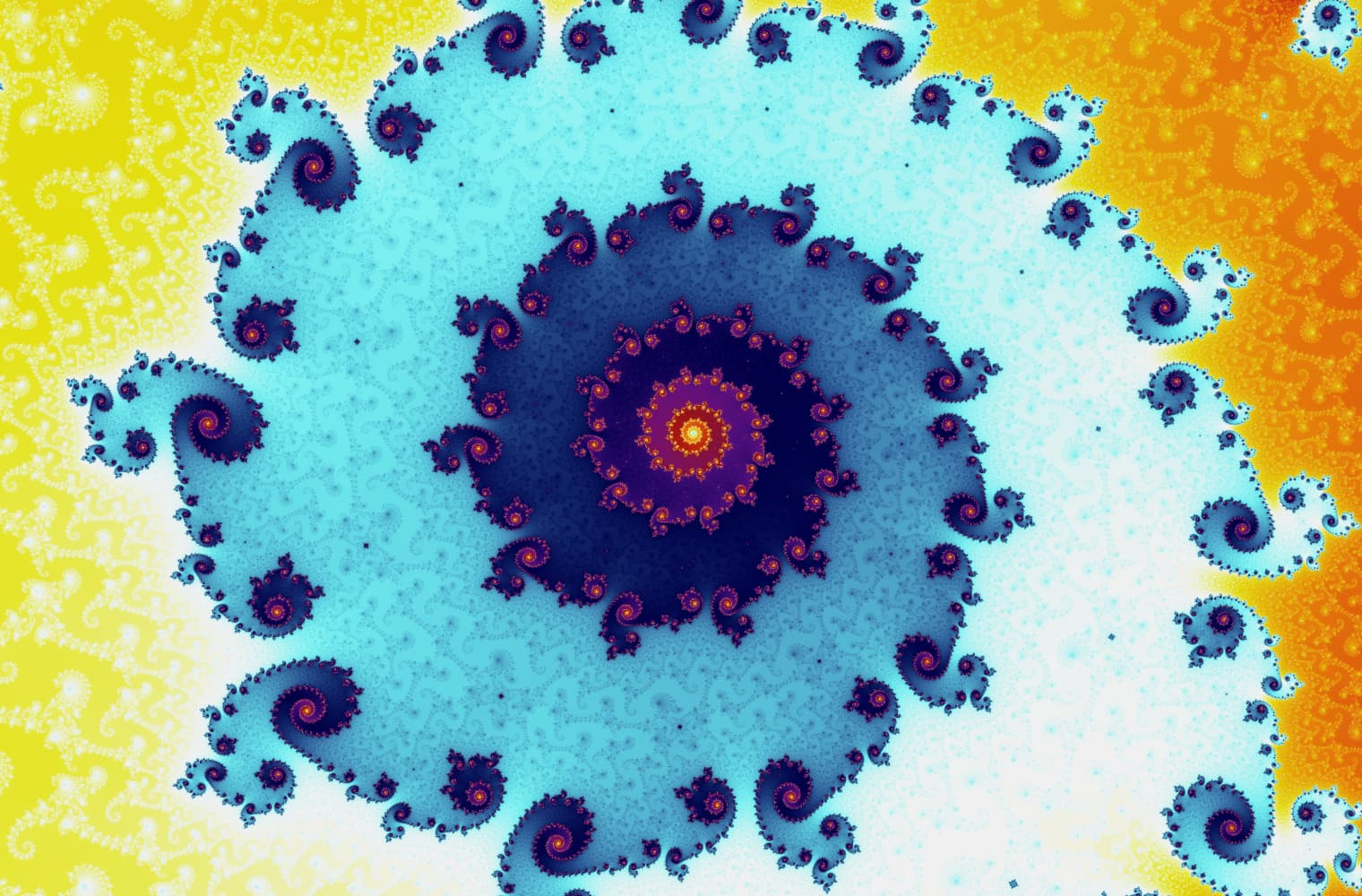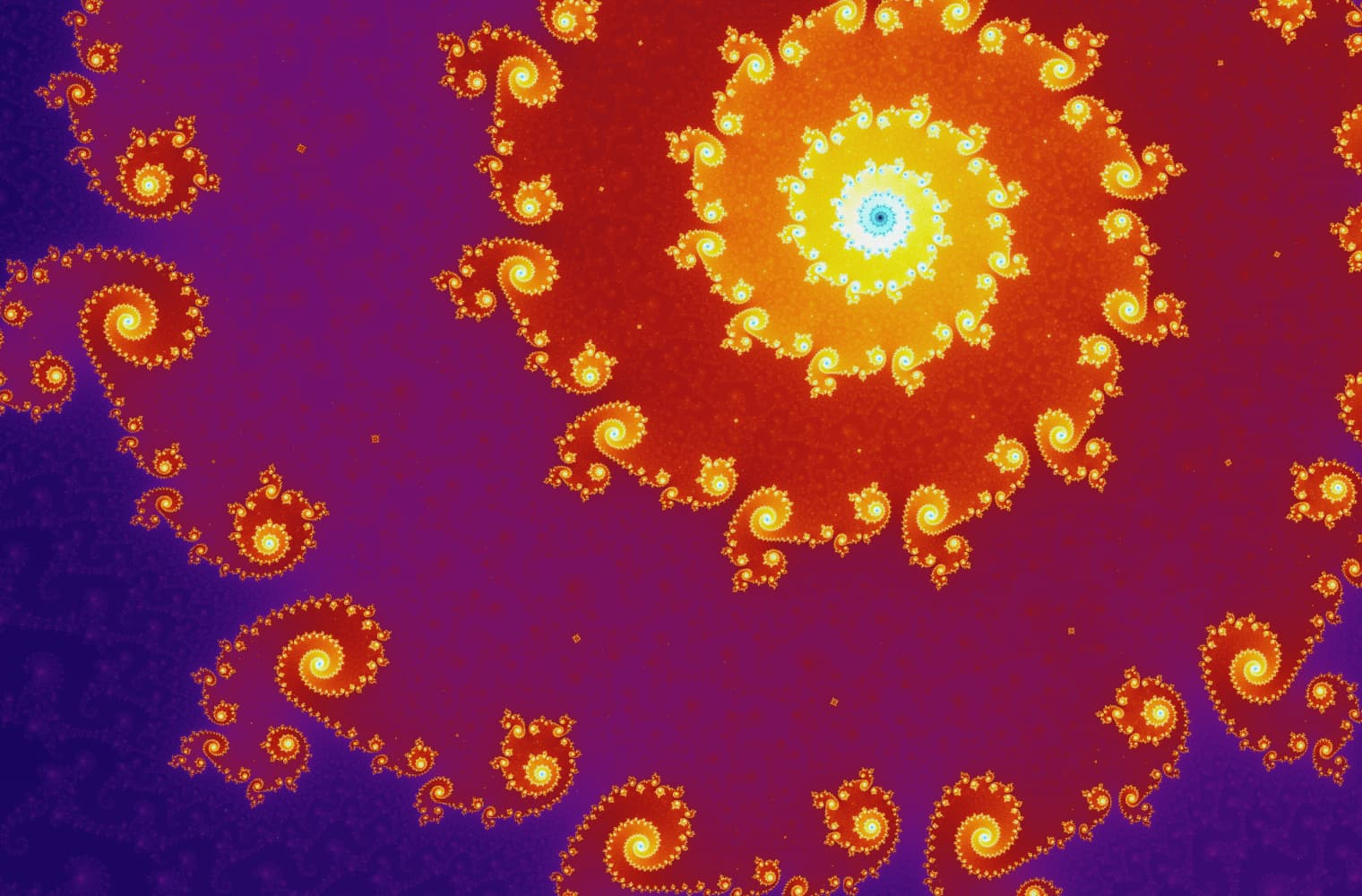Scale: \${pow(scale)}• 曼德布罗特集（Mandelbrot Set）的主体部分内的所有序列都是 到一个点。
• 在顶部球状体内的所有序列 它由个点组成。
• 较小球状体里的序列有一长度为 的轨迹。

1985年，曼德布罗特集（Mandelbrot Set）出现在_《科学美国人》_杂志的封面上，从那时起，它已成为世界上最具识别度的数学图形之一。您可以在T恤、音乐视频和屏幕保护程序中看到它，它也被很多流行书籍和电影引用。

Archie